Related Articles

# Class 9 RD Sharma Solutions – Chapter 11 Coordinate Geometry – Exercise 11.1

• Last Updated : 30 Apr, 2021

### Question 1. Plot the following points on the graph paper:

(i) (2, 5)      (ii) (4, -3)      (iii) (-5, -7)     (iv) (7, -4)     (v) (-3, 2)

(vi) (7, 0)    (vii) (-4, 0)    (viii) (0, 7)      (ix) (0, -4)     (x) (0, 0)

Solution:

Let us considered O (0, 0) be the origin.

(i) Let the given point be A = (2, 5)

Now, the A is at 5 units of distance from x- axis and at 2 units of distance from y-axis.

So, A is in the 1st quadrant.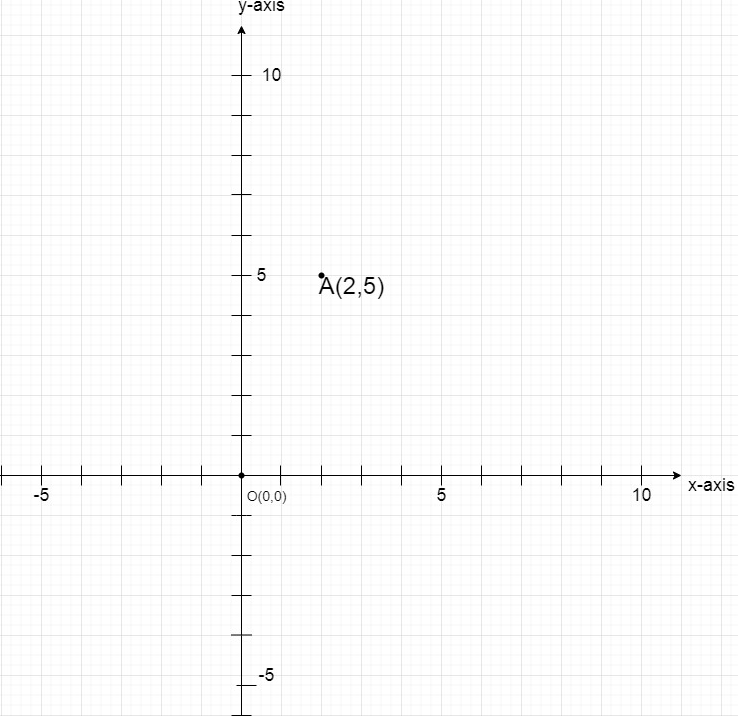(ii) Let the given point be B = (4,-3)

Now, the B is at -3 units of distance from x- axis and at 4 units of distance from y-axis.

So, B is in the 4th quadrant.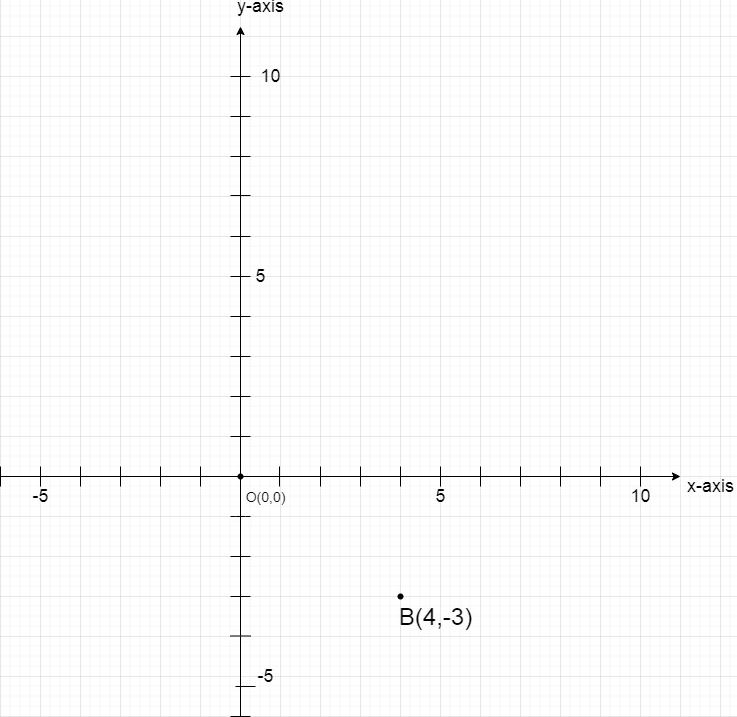(iii) Let the give point be C = (-5, -7)

Now, the C is at -7 units of distance from x- axis and at -5 units of distance from y-axis.

So, C is in the 3rd quadrant.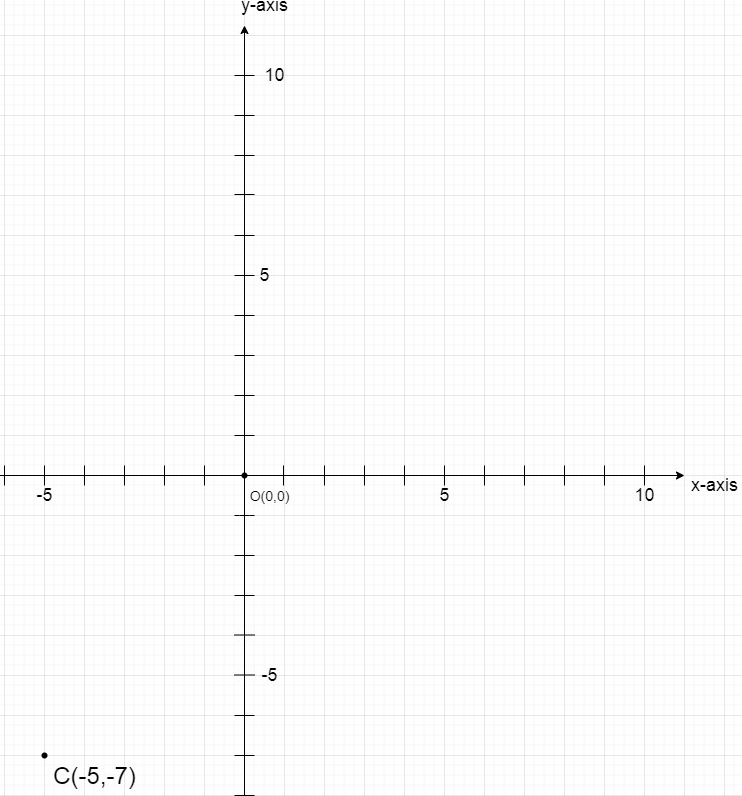(iv) Let the give point be D = (7, -4)

Now, the D is at -4 units of distance from x- axis and at 7 units of distance from y-axis.

So, D is in the 4th quadrant.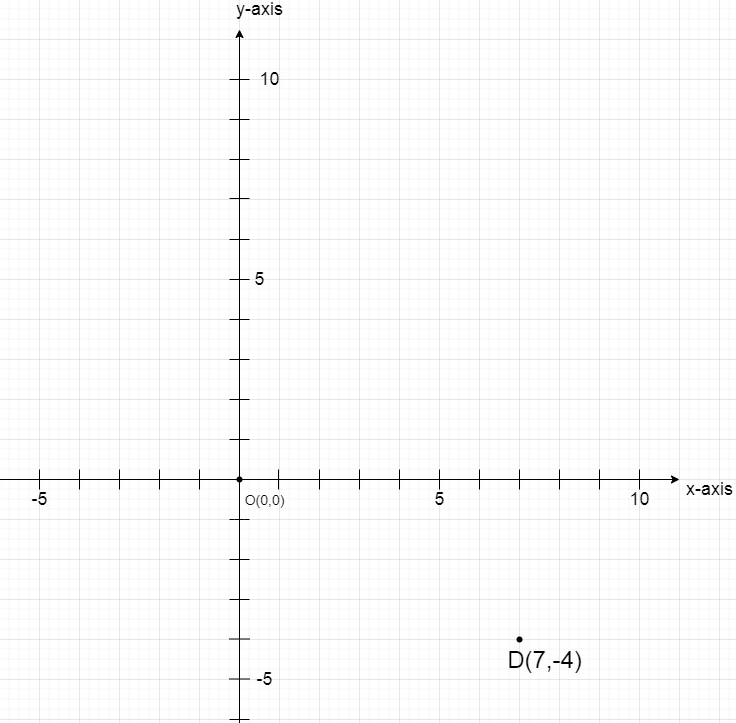(v) Let the give point be E = (-3, 2)

Now, the E is at 2 units of distance from x- axis and at -3 units of distance from y-axis.

So, E is in the 2nd quadrant.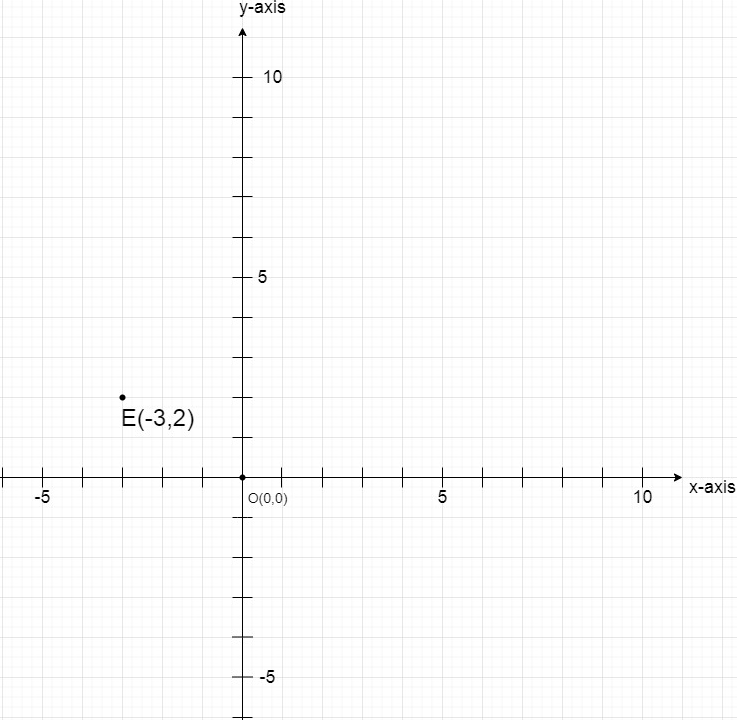(vi) Let the give point be F = (7, 0)

Now, the F is at 0 units of distance from x- axis and at 7 units of distance from y-axis.

So, F is at the positive x-axis.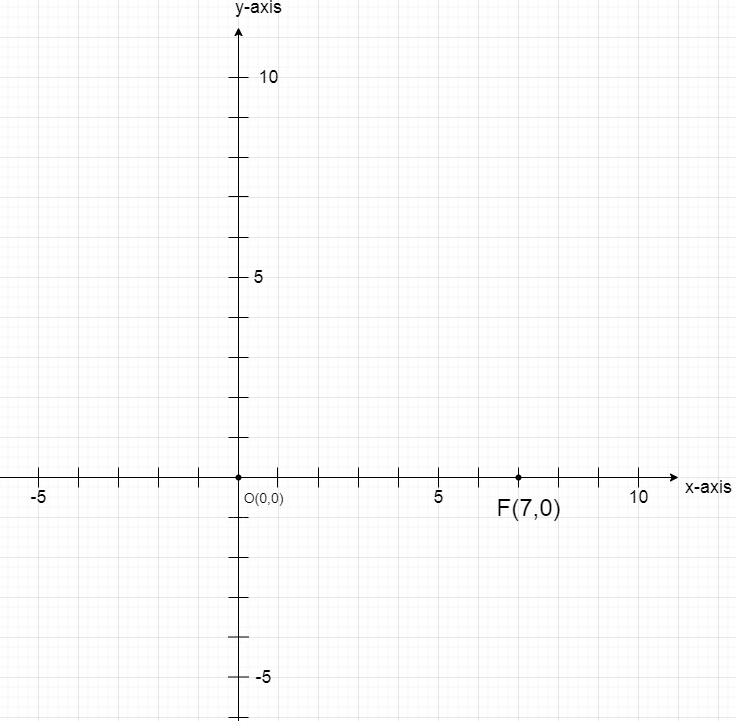(vii) Let the give point be G =(-4, 0)

Now, the G is at 0 units of distance from x- axis and at -4 units of distance from y-axis.

So, G is at the negative x-axis.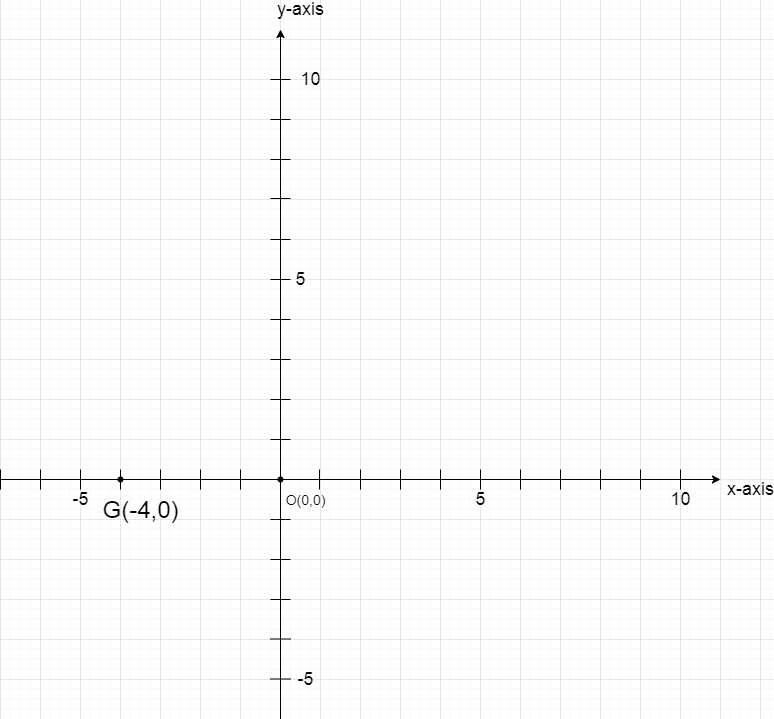(viii) Let the give point be H = (0, 7)

Now, the H is at 0 units of distance from x- axis and at 7 units of distance from y-axis.

So, H is at the positive y-axis.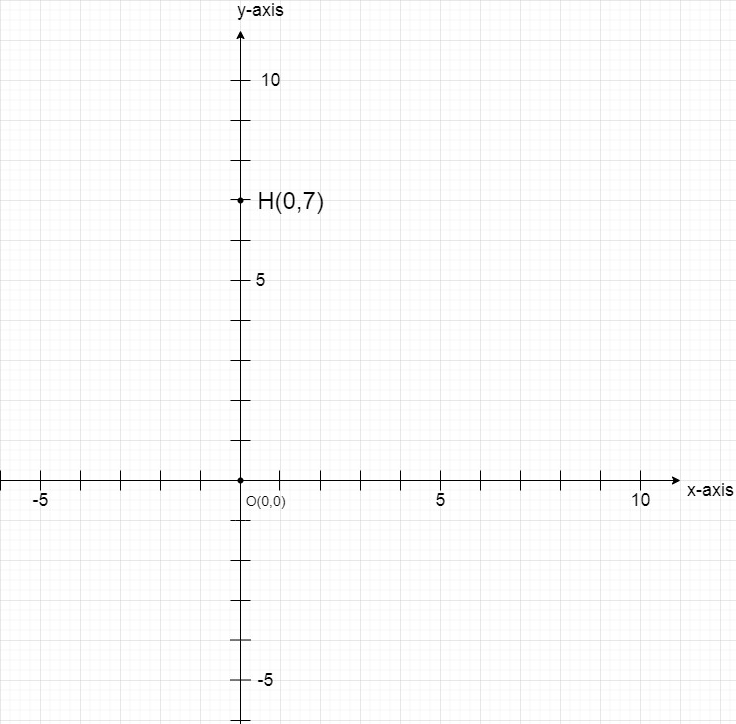(ix) Let the give point be I = (0, -4)

Now, I at -4 units of distance from x- axis and at 0 units of distance from y-axis.

So, I at the negative y-axis.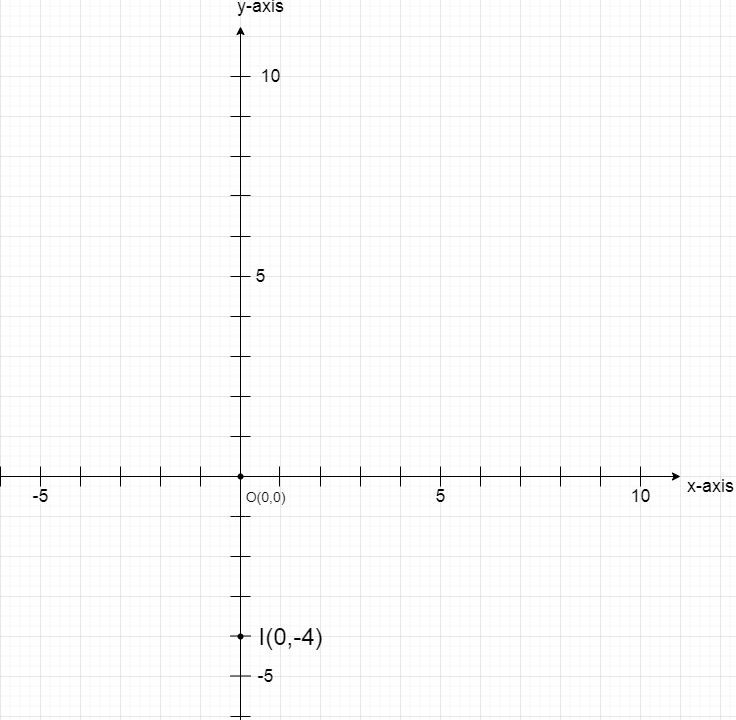(x) Let the give point be J = (0, 0)

Now, the J is at 0 units of distance from x- axis and at 0 units of distance from y-axis.

So, J is the origin only.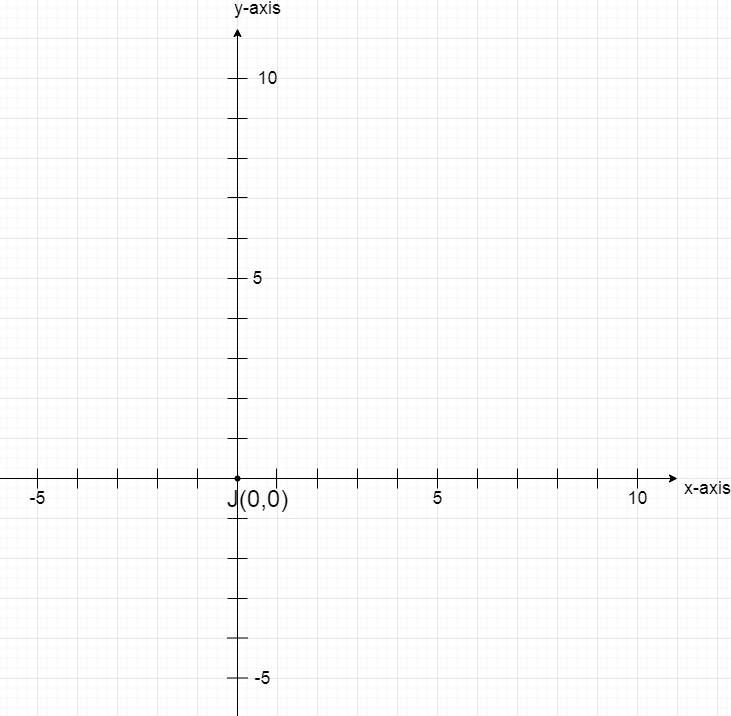### Question 2. Write the coordinates of each of the following points marked in the graph paper.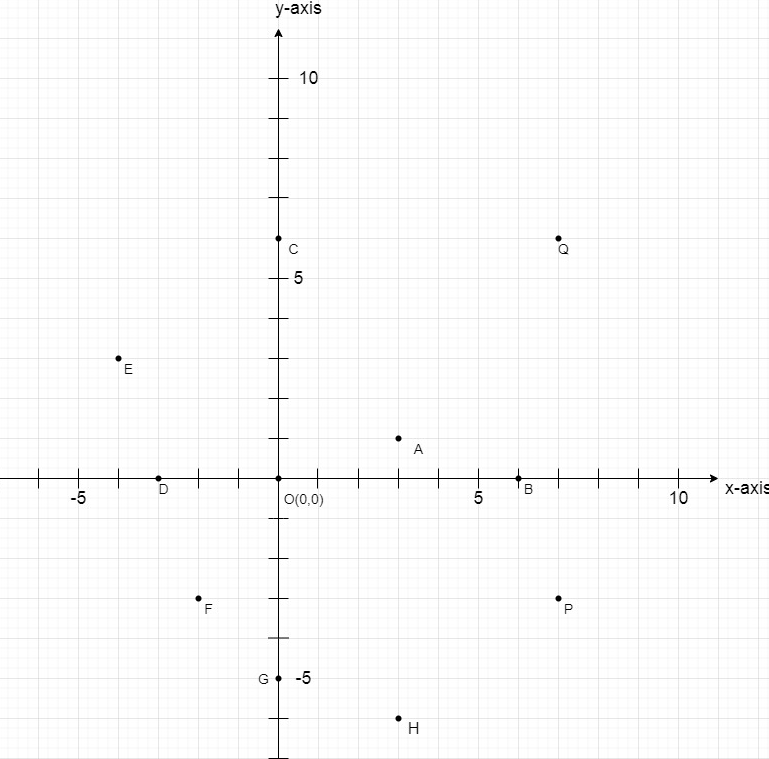Solution:

Here, Point A is at 1 units of distance from x- axis and at 3 units of distance from y-axis.

So, coordinates of point A is (3, 1).

Here, Point B is at 0 units of distance from x- axis and at 6 units of distance from y-axis.

So, coordinates of point B is (6, 0).

Here, Point C is at 6 units of distance from x- axis and at 0 units of distance from y-axis.

So, coordinates of point C is (0, 6).

Here, Point D is at 0 units of distance from x- axis and at -3 units of distance from y-axis.

So, coordinates of point D is (-3, 0).

Here, Point E is at 3 units of distance from x- axis and at -4 units of distance from y-axis.

So, coordinates of point E is (-4, 3).

Here, Point F is at -4 units of distance from x- axis and at -2 units of distance from y-axis.

So, coordinates of point F is (-2, -4).

Here, Point G is at -5 units of distance from x- axis and at 0 units of distance from y-axis.

So, coordinates of point G is (0, -5).

Here, Point H is at -6 units of distance from x- axis and at 3 units of distance from y-axis.

So, coordinates of point H is (3, -6).

Here, Point P is at -3 units of distance from x- axis and at 7 units of distance from y-axis.

So, coordinates of point P is (7, -3).

Here, Point Q is at 6 units of distance from x- axis and at 7 units of distance from y-axis.

So, coordinates of point Q is (7, 6).

Hence, all the points are A(3, 1), B(6, 0), C(0, 6), D(-3, 0), E(-4, 3), F(-2, -4), G(0, -5),

H(3, -6), P(7, -3) and Q(7, 6).

Attention reader! All those who say programming isn’t for kids, just haven’t met the right mentors yet. Join the  Demo Class for First Step to Coding Coursespecifically designed for students of class 8 to 12.

The students will get to learn more about the world of programming in these free classes which will definitely help them in making a wise career choice in the future.

My Personal Notes arrow_drop_up